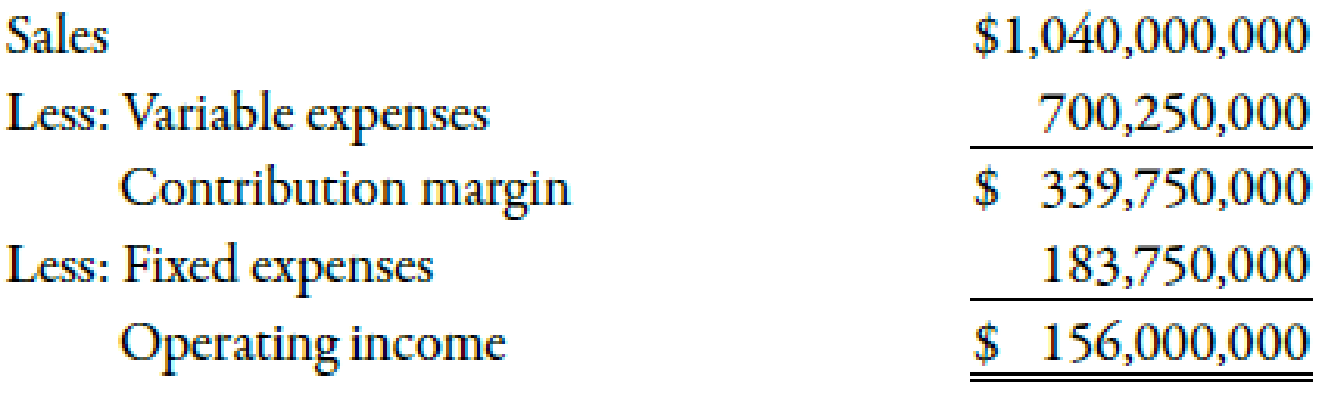Chapter 11, Problem 27E### Managerial Accounting: The Corners...

7th Edition
Maryanne M. Mowen + 2 others
ISBN: 9781337115773

#### Solutions

Chapter
Section### Managerial Accounting: The Corners...

7th Edition
Maryanne M. Mowen + 2 others
ISBN: 9781337115773
Textbook Problem
20 views

# Margin, Turnover, Return on Investment, Average Operating AssetsElway Company provided the following income statement for the last year:At the beginning of last year, Elway had $28,300,000 in operating assets. At the end of the year, Elway had$23,700,000 in operating assets.Required: 1. Compute average operating assets. 2. Compute the margin and turnover ratios for last year. (Note: Round the answer for margin ratio to two decimal places.) 3. Compute ROI. (Note: Round answer to two decimal places.) 4. CONCEPTUAL CONNECTION Briefly explain the meaning of ROI. 5. CONCEPTUAL CONNECTION Comment on why the ROI for Elway Company is relatively high (as compared to the lower ROI of a typical manufacturing company).

1.

To determine

Calculate the average operating income.

Explanation

Average Operating Assets:

Average operating assets can be determined by adding the beginning assets and ending assets and dividing the result by 2.

Use the following formula to calculate average operating assets:

Average operating assets=(Beginning assets+Ending assets)2

Substitute $28,300,000 for beginning assets and$23,700,000 for ending assets in the above formula

2.

To determine

Calculate margin and turnover ratios.

3.

To determine

Calculate ROI.

4.

To determine

Describe ROI.

5.

To determine

Describe the reason that the ROI of Company E is relatively high.

### Still sussing out bartleby?

Check out a sample textbook solution.

See a sample solution

#### The Solution to Your Study Problems

Bartleby provides explanations to thousands of textbook problems written by our experts, many with advanced degrees!

Get Started